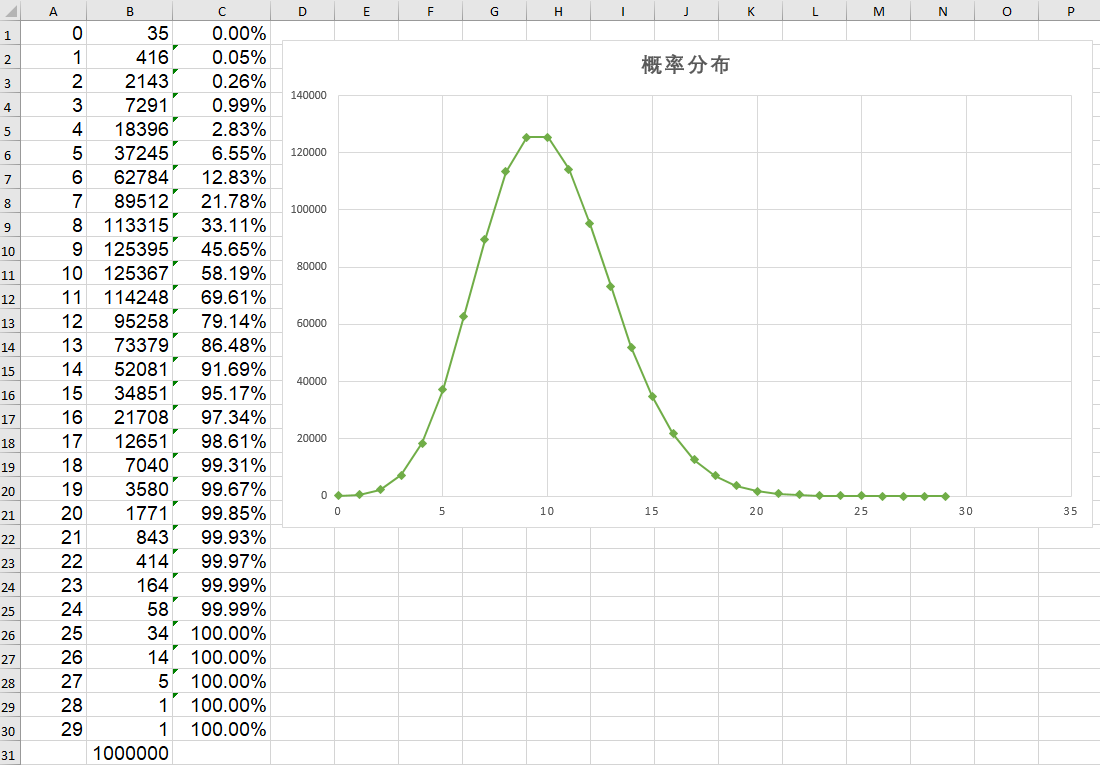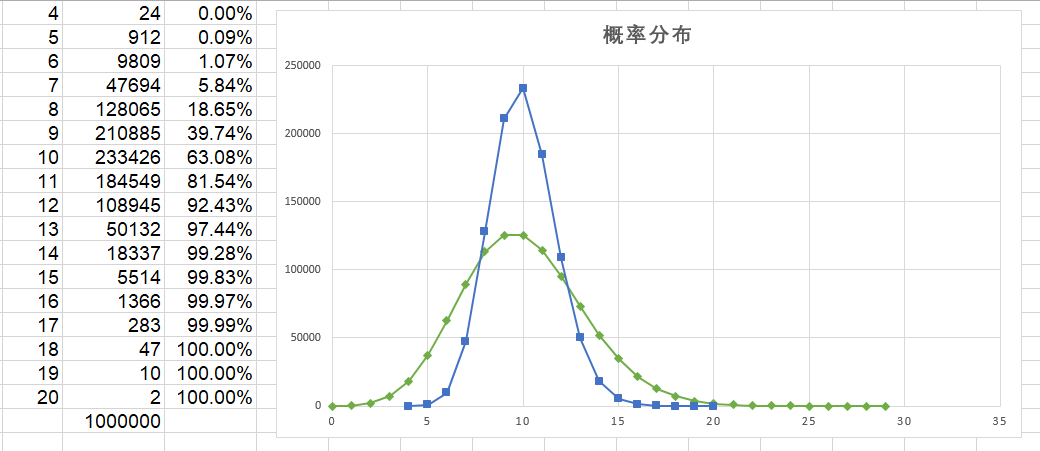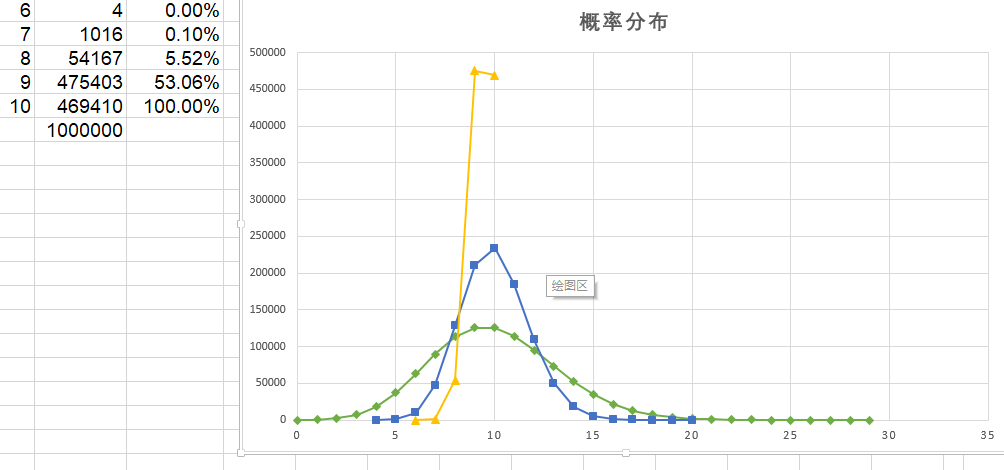# 随机``````math.randomseed(os.time())

local times1 = 1000000
local times2 = 1000

local percent = 0.01

local result = {}
for i = 1,times1 do
local t = 0
for j=1,times2 do
local p = math.random(math.maxinteger) / math.maxinteger
if p < percent then
t=t+1
end
end
result[t] = (result[t] or 0) + 1
end
``````
Written on October 22, 2018